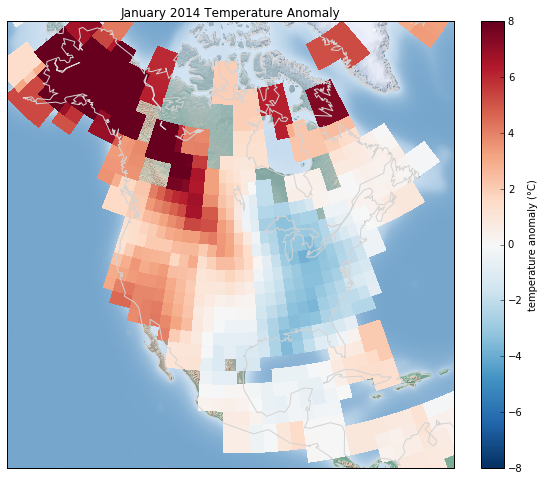# 8.16 地理数据和 Basemap

Basemap 的安装很简单；如果你正在使用 conda，你可以输入这个，然后下载包：

$conda install basemap  我们只在标准样板中添加一个新的导入： %matplotlib inline import numpy as np import matplotlib.pyplot as plt from mpl_toolkits.basemap import Basemap  一旦安装并导入了 Basemap 工具包，地理绘图就能在几行之内实现（下面的图形也需要 Python 2 中的PIL包，或者 Python 3 中的pillow包）： plt.figure(figsize=(8, 8)) m = Basemap(projection='ortho', resolution=None, lat_0=50, lon_0=-100) m.bluemarble(scale=0.5);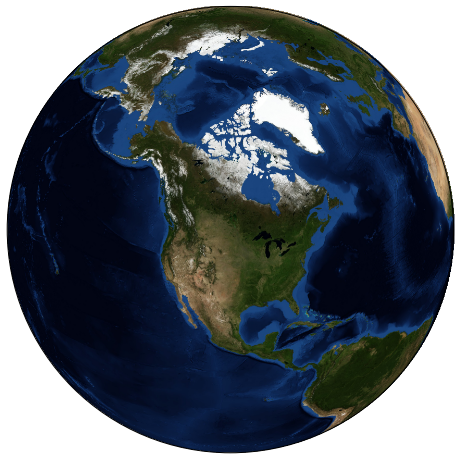Basemap参数的含义将立即讨论。 有用的是这里显示的地球不仅仅是一个图像; 它是一个功能齐全的 Matplotlib 轴域，它可以理解球面坐标，这使我们可以轻松地在地图上绘制数据！例如，我们可以使用不同的地图投影，放大到北美并绘制西雅图的位置。我们将使用 etopo 图像（显示陆地和海底的地形特征）作为地图背景： fig = plt.figure(figsize=(8, 8)) m = Basemap(projection='lcc', resolution=None, width=8E6, height=8E6, lat_0=45, lon_0=-100,) m.etopo(scale=0.5, alpha=0.5) # 将 (long, lat) 映射为 (x, y) 以便绘图 x, y = m(-122.3, 47.6) plt.plot(x, y, 'ok', markersize=5) plt.text(x, y, ' Seattle', fontsize=12);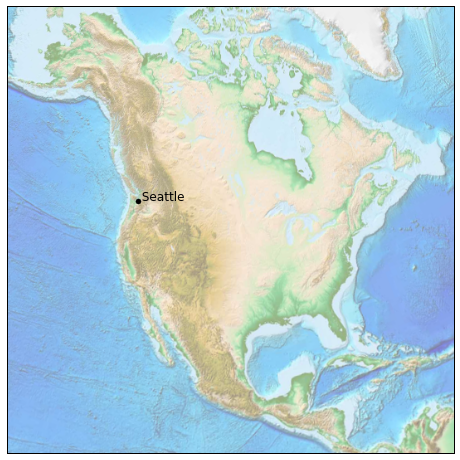通过几行 Python，你可以轻松了解可能的地理可视化类型。我们现在将更深入地讨论 Basemap 的功能，并提供几个可视化地图数据的示例。使用这些简短的示例作为积木，你应该能够创建几乎任何你想要的地图可视化。 ## 地图投影 使用地图时要决定的第一件事，是要使用什么投影。你可能已经熟悉这样一个事实：不可能将球形地图（例如地球的地图）投影到平坦的表面上，而不会以某种方式扭曲或破坏其连续性。这些预测是在人类历史进程中发展起来的，有很多选择！取决于地图投影的预期用途，有一些地图特征，保留它们很有用（例如，方向，区域，距离，形状或其他考虑因素）。 Basemap 包实现了几十个这样的投影，全部由短格式代码引用。在这里，我们将简要介绍一些比较常见的。我们首先定义一个便利例程来绘制我们的世界地图以及经纬线： from itertools import chain def draw_map(m, scale=0.2): # 绘制阴影浮雕图像 m.shadedrelief(scale=scale) # 将 lats 和 lons 作为字典返回 lats = m.drawparallels(np.linspace(-90, 90, 13)) lons = m.drawmeridians(np.linspace(-180, 180, 13)) # 键包含 plt.Line2D 实例 lat_lines = chain(*(tup for tup in lats.items())) lon_lines = chain(*(tup for tup in lons.items())) all_lines = chain(lat_lines, lon_lines) # 遍历这些线并设置所需的样式 for line in all_lines: line.set(linestyle='-', alpha=0.3, color='w')  ### 圆柱投影 最简单的地图投影是圆柱投影，其中恒定纬度和经度的线分别映射到水平线和垂直线。这种类型的映射很好地代表了赤道区域，但产生了极点附近的极端扭曲。纬线的间距在不同的圆柱投影之间变化，产生不同的保留特征，并且在极点附近的不同的变形。在下图中，我们展示了等距圆柱投影的示例，它选择了沿子午线保留距离的纬度缩放。其他圆柱投影是墨卡托（projection='merc'）和圆柱等积（projection='cea'）投影。 fig = plt.figure(figsize=(8, 6), edgecolor='w') m = Basemap(projection='cyl', resolution=None, llcrnrlat=-90, urcrnrlat=90, llcrnrlon=-180, urcrnrlon=180, ) draw_map(m)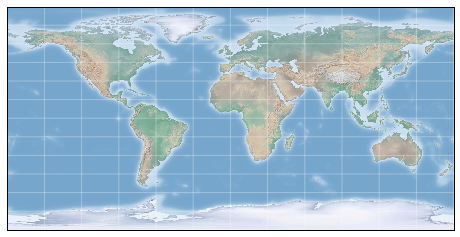此视图的 Basemap 的附加参数，为所需的地图指定左下角（llcrnr）和右上角（urcrnr）的纬度（lat）和经度（lon），以度为单位。 ### 伪圆柱投影 伪圆柱投影放松了子午线（恒定经线）保持垂直的要求; 这可以在投影的极点附近提供更好的特性。墨卡托投影（projection ='moll'）是这方面的一个常见例子，其中所有经线都是椭圆弧。它的构造是为了保留地图上的区域：尽管两极附近存在扭曲，但小块的区域反映了真实区域。其他伪圆柱投影是正弦曲线（projection='sinu'）和罗宾逊（projection='robin'）投影。 fig = plt.figure(figsize=(8, 6), edgecolor='w') m = Basemap(projection='moll', resolution=None, lat_0=0, lon_0=0) draw_map(m)这里 Basemap 的额外参数是指所需映射的中心纬度（lat_0）和经度（lon_0）。 ### 透视投影 透视投影使用透视点的特定选择构建，类似于你从空间中的特定点拍摄地球（对于某些投影，技术上点位于地球内部！）。一个常见的例子是正交投影（projection='ortho'），它显示了远处的观察者看到的地球的一侧。因此，它一次只能显示全球的一半。 其他基于视角的投影包括 gnomonic 投影（projection='gnom'）和立体投影（projection='stere'）。这些通常对于显示地图的一小部分最有用。 以下是正交投影的示例： fig = plt.figure(figsize=(8, 8)) m = Basemap(projection='ortho', resolution=None, lat_0=50, lon_0=0) draw_map(m);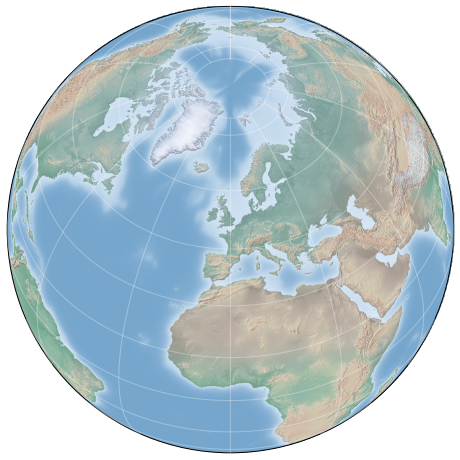### 圆锥投影 圆锥投影将地图投影到单个圆锥上，然后展开。这可以产生非常好的局部特性，但是远离圆锥焦点的区域可能变得非常扭曲。其中一个例子是 Lambert Conformal 圆锥投影（projection='lcc'），我们之前在北美地图中看到过。 它将地图投影到一个圆锥上，这个圆锥的排列方式使得两个标准平行线（在 Basemap 中由lat_1lat_2规定）的距离是良好表示的，比例在它们之间减小并且在它们之外增加。其他有用的圆锥投影是等距圆锥投影（projection='eqdc'）和 Albers 等面投影（projection='aea'）。圆锥投影，就像透视投影，往往是表示地球中小块区域的良好选择。 fig = plt.figure(figsize=(8, 8)) m = Basemap(projection='lcc', resolution=None, lon_0=0, lat_0=50, lat_1=45, lat_2=55, width=1.6E7, height=1.2E7) draw_map(m)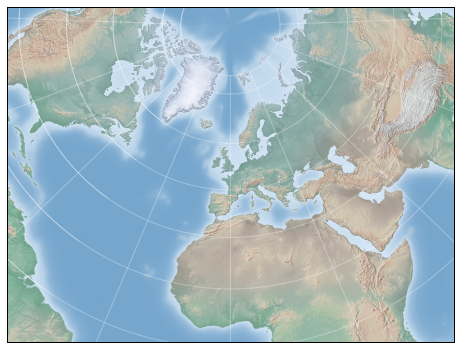### 其它投影 如果你要对基于地图的可视化做很多事情，我建议你了解其他可用的投影，以及它们的属性，优点和缺点。 最有可能的是，它们可以在 Basemap 包中找到。 如果你深入研究这个主题，你会发现一个令人难以置信的极客的亚文化 geo-viz，他们为热烈争论做好了准备，来为任何给定应用支持他们最喜欢的投影！ ## 绘制地图背景 之前我们看过bluemarble()shadedrelief()方法，用于在地图上投影全球图像，以及drawparallels()drawmeridians()方法用于绘制恒定经纬度的线。Basemap 包包含一系列有用的函数，用于绘制物理特征的边界，例如大陆，海洋，湖泊和河流等，以及政治边界，例如国家地区，以及美国各州和县。以下是一些可用绘图功能，你可能希望使用 IPython 帮助特性来探索： • 物理边界和水体 • drawcoastlines()：绘制大陆海岸线 • drawlsmask()：绘制陆地和海洋之间的掩码，用于在一个或另一个上投射图像 • drawmapboundary()：绘制地图边界，包括海洋的填充颜色。 • drawrivers()：在地图上绘制河流 • fillcontinents()：用给定的颜色填充大陆；可选择用另一种颜色填充湖泊 • 政治边界 • drawcountries()：绘制国界 • drawstates()：绘制美国国界 • drawcounties()：绘制美国县界 • 地图功能 • drawgreatcircle()：在两点之间绘制大圆圈 • drawparallels()：绘制恒定纬度的线条 • drawmeridians()：绘制恒定经度的线条 • drawmapscale()：在地图上绘制线性刻度 • 全球图像 • bluemarble()：将 NASA 的蓝色大理石图像投影到地图上 • shadedrelief()：将阴影浮雕图像投影到地图上 • etopo()：在地图上绘制一个 etopo 浮雕图像 • warpimage()：将用户提供的图像投影到地图上 对于基于边界的特性，必须在创建 Basemap 图像时设置所需的分辨率。Basemap类的resolution参数设置边界中的细节级别，他们是'c'（原始），'l'（低），'i'（中），'h'（高），'f'（完整）或None（如果没有使用边界）。这个选项很重要：例如，在全局地图上设置高分辨率边界可能非常慢。 这是绘制陆地/海洋边界，以及分辨率参数的效果的示例。我们将创建苏格兰的美丽的斯凯岛的低分辨率和高分辨率地图。它位于北纬 57.3°，6.2°W，90,000×120,000 公里的地图很好显示它： fig, ax = plt.subplots(1, 2, figsize=(12, 8)) for i, res in enumerate(['l', 'h']): m = Basemap(projection='gnom', lat_0=57.3, lon_0=-6.2, width=90000, height=120000, resolution=res, ax=ax[i]) m.fillcontinents(color="#FFDDCC", lake_color='#DDEEFF') m.drawmapboundary(fill_color="#DDEEFF") m.drawcoastlines() ax[i].set_title("resolution='{0}'".format(res));请注意，低分辨率海岸线不适合此级别的缩放，而高分辨率的工作正常。然而，低水平对于全局视图来说效果会很好，并且比加载整个地球的高分辨率边界数据要快得多！可能需要进行一些实验，才能找到给定视图的正确分辨率参数：最佳路径是从快速低分辨率的绘图开始，并根据需要增加分辨率。 ## 在 Basemap 上绘制数据 也许 Basemap 工具包中最有用的部分，是将各种数据绘制到地图背景上的能力。对于简单的绘图和文本，任何plt函数都可以在地图上执行；你可以使用Basemap实例将纬度和经度坐标投影到(x, y)坐标，用于plt的绘图，正如我们在西雅图示例中所见。 除此之外，还有许多特定于地图的函数，可用作Basemap实例的方法。这些东西与它们的标准 Matplotlib 对应物非常相似，但是有一个额外的布尔参数latlon，如果设置为True，它允许你将原始纬度和经度传递给方法，而不是投影(x, y)坐标。 其中一些特定于地图的方法是： • contour()/contourf()：绘制等高线或填充的等高线 • imshow()：绘制图像 • pcolor()/pcolormesh()：为不规则/规则网格绘制伪彩色图 • plot()：绘制线条和/或标记。 • scatter()：绘制带标记的点。 • quiver()：绘制向量。 • barbs()：绘制风向。 • drawgreatcircle()：绘制大圆圈。 我们将继续并看到其中一些例子。这些函数的更多信息，包括几个示例图，请参阅在线 Basemap 文档 ## 示例：加利福尼亚的城市 回想一下，在“自定义图例”中，我们演示了在散点图中使用大小和颜色，来传达加州城市的位置，大小和人口的信息。在这里，我们将再次创建此绘图，但使用 Basemap 将数据放在上下文中。 我们开始加载数据，就像我们之前做的那样： import pandas as pd cities = pd.read_csv('data/california_cities.csv') # 提取我们感兴趣的数据 lat = cities['latd'].values lon = cities['longd'].values population = cities['population_total'].values area = cities['area_total_km2'].values  接下来，我们设置地图投影，绘制数据的散点图，然后创建颜色条和图例： # 1. 绘制地图北京 fig = plt.figure(figsize=(8, 8)) m = Basemap(projection='lcc', resolution='h', lat_0=37.5, lon_0=-119, width=1E6, height=1.2E6) m.shadedrelief() m.drawcoastlines(color='gray') m.drawcountries(color='gray') m.drawstates(color='gray') # 2. 绘制城市数据的散点图，其中颜色反映人口 # 尺寸反映面积 m.scatter(lon, lat, latlon=True, c=np.log10(population), s=area, cmap='Reds', alpha=0.5) # 3. 创建颜色条和图例 plt.colorbar(label=r'$\log_{10}({\rm population})$') plt.clim(3, 7) # 使用虚拟的点生成图例 for a in [100, 300, 500]: plt.scatter([], [], c='k', alpha=0.5, s=a, label=str(a) + ' km$^2$') plt.legend(scatterpoints=1, frameon=False, labelspacing=1, loc='lower left');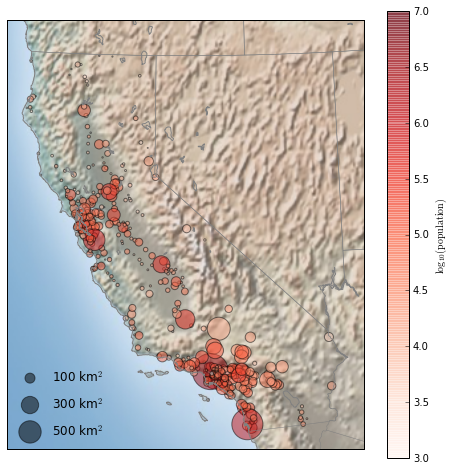这向我们展示了大量人口在加利福尼亚定居的地方：它们聚集在洛杉矶和旧金山地区的海岸附近，沿着平坦的中央山谷中的高速公路延伸，几乎完全避开了沿着国界的山区。 ## 示例：表面温度数据 作为一些更连续的地理数据的可视化示例，让我们考虑一下 2014 年 1 月袭击美国东部的“极涡”。任何气候数据的重要来源是美国宇航局 Goddard 空间研究所。这里我们将使用 GIS 250 温度数据，我们可以使用 shell 命令下载（在 Windows 机器上，这些命令可能必须修改）。此处使用的数据下载于 2016 年 6 月 12 日，文件大小约为 9MB： # !curl -O http://data.giss.nasa.gov/pub/gistemp/gistemp250.nc.gz # !gunzip gistemp250.nc.gz  数据采用NetCDF格式，可以通过netCDF4库在 Python 中读取。你可以像此处所示安装此库： $ conda install netcdf4


from netCDF4 import Dataset
data = Dataset('gistemp250.nc')


from netCDF4 import date2index
from datetime import datetime
timeindex = date2index(datetime(2014, 1, 15),
data.variables['time'])


lat = data.variables['lat'][:]
lon = data.variables['lon'][:]
lon, lat = np.meshgrid(lon, lat)
temp_anomaly = data.variables['tempanomaly'][timeindex]


fig = plt.figure(figsize=(10, 8))
m = Basemap(projection='lcc', resolution='c',
width=8E6, height=8E6,
lat_0=45, lon_0=-100,)
m.pcolormesh(lon, lat, temp_anomaly,
latlon=True, cmap='RdBu_r')
plt.clim(-8, 8)
m.drawcoastlines(color='lightgray')

plt.title('January 2014 Temperature Anomaly')
plt.colorbar(label='temperature anomaly (°C)');
`